Checkout JEE MAINS 2022 Question Paper Analysis : Checkout JEE MAINS 2022 Question Paper Analysis :

# FAQ’s on Toppling

Toppling is a phenomenon that generally happens when force is applied to a body of the smaller base area. We will discuss this topic with the help of a few questions.

## Important Questions For Toppling

• With the help of an example define toppling?

Ans – Suppose we have a block as shown in the figure, when we apply force there should be translational motion.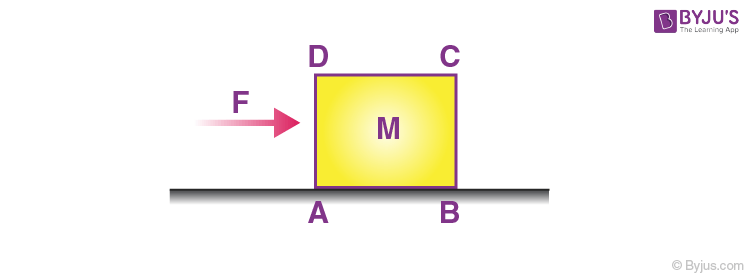But sometimes instead of translational motion, the body rotates about an axis passing through the rightmost edge. The box topples over to take the position as shown below.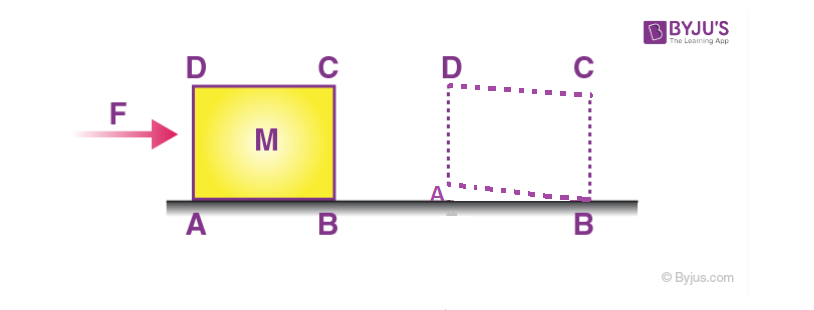• Explain with the help of FBD what happens during toppling?

Ans – Suppose the force is applied on the body as shown below,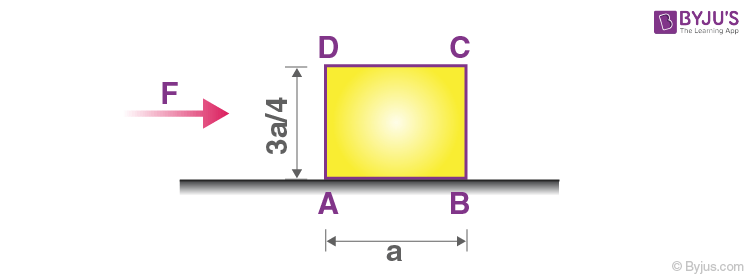In order to counter the torque caused by F about the centre of mass the normal will shift.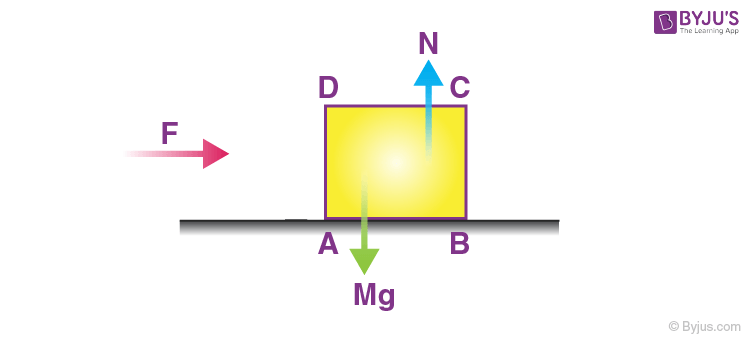We have to find the maximum force that can be applied so that the block does not topple, in that case, the normal will pass through the extreme right corner.

Taking torque about an axis passing through the rightmost corner,

mga/2 = F . 3a/4

or F =(2/3) mg

So if the force is greater than this the block topples over.

• A force is applied on the block as shown. We have to find whether it topples first or starts moving. Assume the coefficient of friction between the box and the surface is 0.5.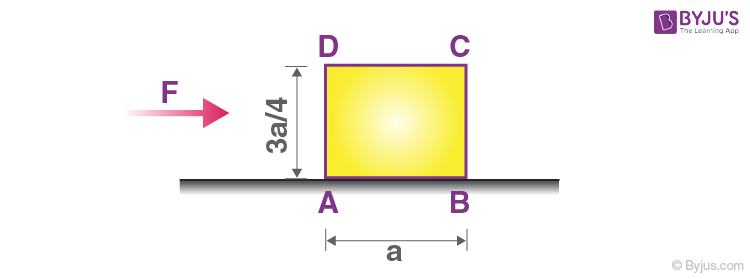Ans – The first step is to draw the FBD,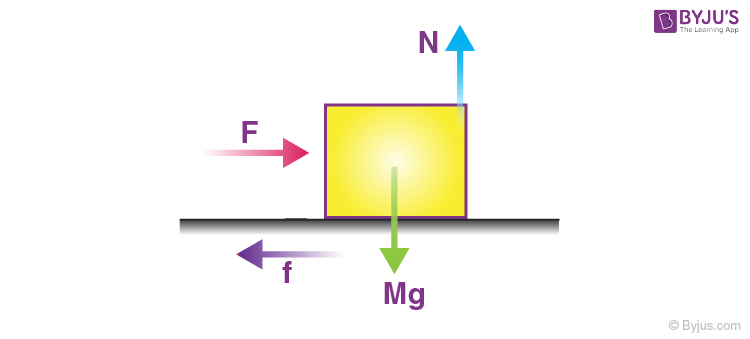From vertical equilibrium,

$$\begin{array}{l}N~=~Mg\end{array}$$
—- (1)

$$\begin{array}{l}f_s\end{array}$$
=
$$\begin{array}{l}0.5~ Mg\end{array}$$
—- (2)

For the case when toppling is about to occur,

Mga/2 = F . 3a/4

or F = 2/3 Mg

or F = 0.667 Mg …(3)

Comparing (2) and (3) we get,

On increasing the force the body first starts moving and when it reaches 0.66 Mg the block tends to topple over.